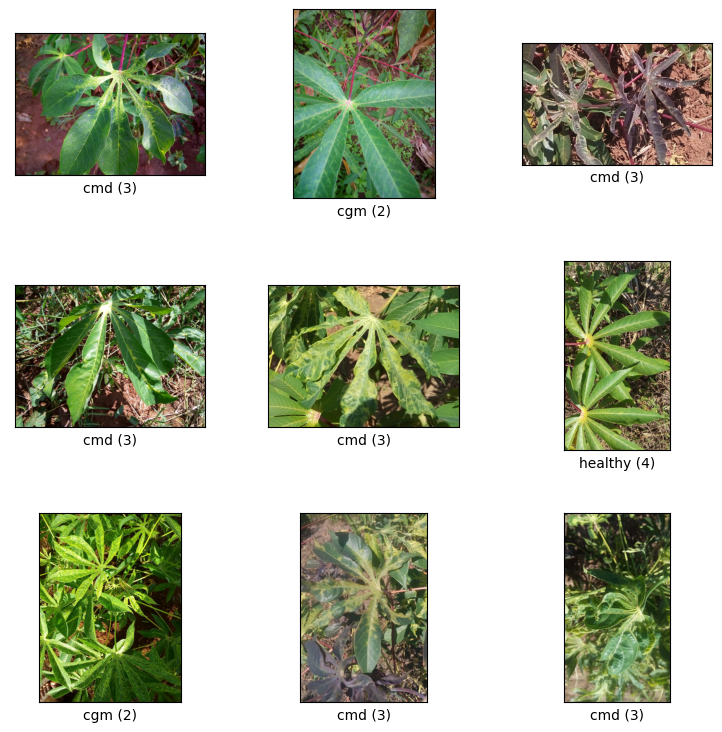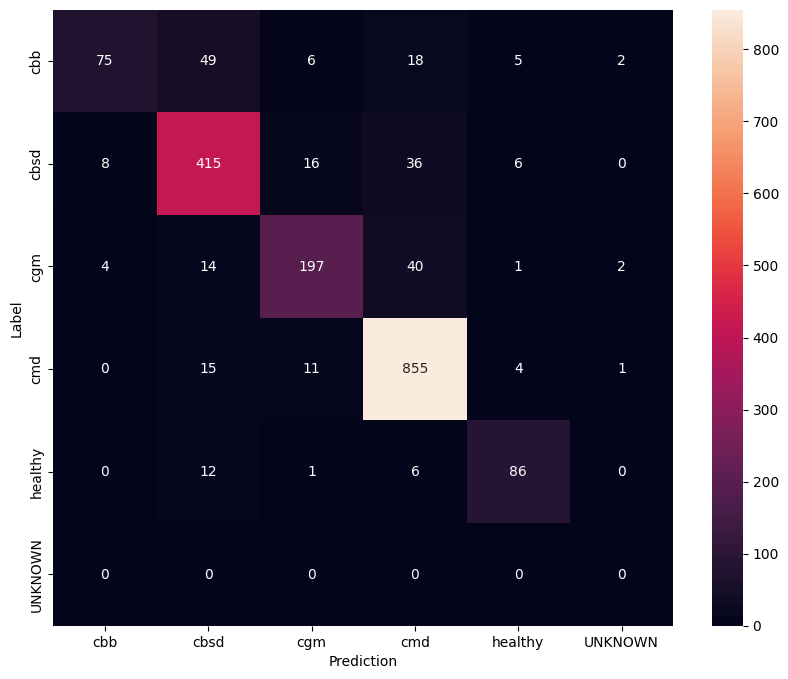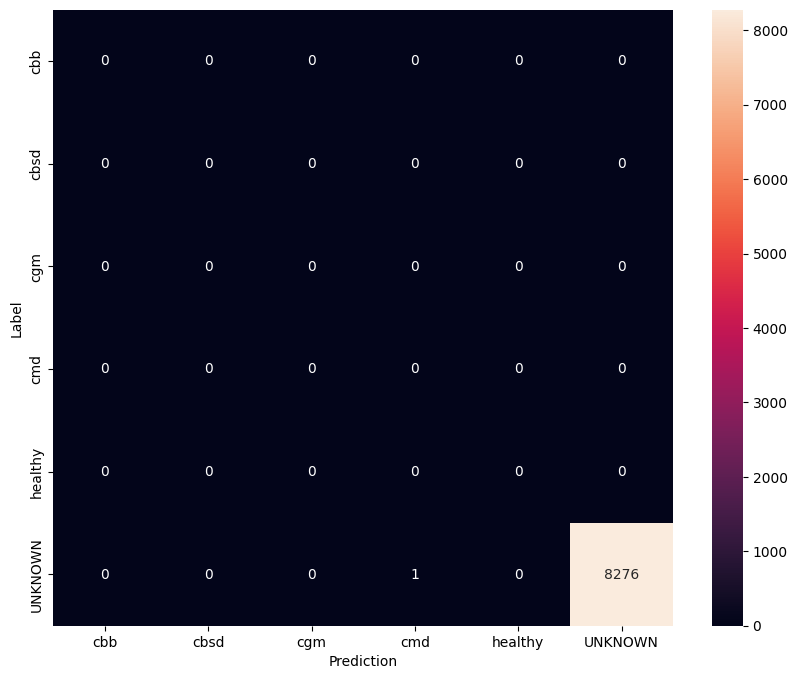# Fine tuning models for plant disease detection

Stay organized with collections Save and categorize content based on your preferences.

This notebook shows you how to fine-tune CropNet models from TensorFlow Hub on a dataset from TFDS or your own crop disease detection dataset.

You will:

• Enrich the data with unknown (negative) examples to get a more robust model
• Apply image augmentations to the data
• Load and fine tune a CropNet model from TF Hub
• Export a TFLite model, ready to be deployed on your app with Task Library, MLKit or TFLite directly

## Imports and Dependencies

Before starting, you'll need to install some of the dependencies that will be needed like Model Maker and the latest version of TensorFlow Datasets.

````sudo apt install -q libportaudio2`
`## image_classifier library requires numpy <= 1.23.5`
`pip install "numpy<=1.23.5"`
`pip install --use-deprecated=legacy-resolver tflite-model-maker-nightly`
`pip install -U tensorflow-datasets`
`## scann library requires tensorflow < 2.9.0`
`pip install "tensorflow<2.9.0"`
`## tensorflowjs requires packaging < 20.10`
`pip install "packaging<20.10"`
```
``````import matplotlib.pyplot as plt
import os
import seaborn as sns

import tensorflow as tf
import tensorflow_datasets as tfds

from tensorflow_examples.lite.model_maker.core.export_format import ExportFormat

from tflite_model_maker import image_classifier
from tflite_model_maker.image_classifier import ModelSpec
``````
```/tmpfs/src/tf_docs_env/lib/python3.9/site-packages/tensorflow_addons/utils/ensure_tf_install.py:53: UserWarning: Tensorflow Addons supports using Python ops for all Tensorflow versions above or equal to 2.9.0 and strictly below 2.12.0 (nightly versions are not supported).
The versions of TensorFlow you are currently using is 2.8.4 and is not supported.
Some things might work, some things might not.
If you were to encounter a bug, do not file an issue.
If you want to make sure you're using a tested and supported configuration, either change the TensorFlow version or the TensorFlow Addons's version.
warnings.warn(
```

## Load a TFDS dataset to fine-tune on

Lets use the publicly available Cassava Leaf Disease dataset from TFDS.

``````tfds_name = 'cassava'
(ds_train, ds_validation, ds_test), ds_info = tfds.load(
name=tfds_name,
split=['train', 'validation', 'test'],
with_info=True,
as_supervised=True)
TFLITE_NAME_PREFIX = tfds_name
``````

Instead of using a TFDS dataset, you can also train on your own data. This code snippet shows how to load your own custom dataset. See this link for the supported structure of the data. An example is provided here using the publicly available Cassava Leaf Disease dataset.

``````# data_root_dir = tf.keras.utils.get_file(
#     'cassavaleafdata.zip',
#     extract=True)
# data_root_dir = os.path.splitext(data_root_dir)  # Remove the .zip extension

# builder = tfds.ImageFolder(data_root_dir)

# ds_info = builder.info
# ds_train = builder.as_dataset(split='train', as_supervised=True)
# ds_validation = builder.as_dataset(split='validation', as_supervised=True)
# ds_test = builder.as_dataset(split='test', as_supervised=True)
``````

## Visualize samples from train split

Let's take a look at some examples from the dataset including the class id and the class name for the image samples and their labels.

``````_ = tfds.show_examples(ds_train, ds_info)
``````## Add images to be used as Unknown examples from TFDS datasets

Add additional unknown (negative) examples to the training dataset and assign a new unknown class label number to them. The goal is to have a model that, when used in practice (e.g. in the field), has the option of predicting "Unknown" when it sees something unexpected.

Below you can see a list of datasets that will be used to sample the additional unknown imagery. It includes 3 completely different datasets to increase diversity. One of them is a beans leaf disease dataset, so that the model has exposure to diseased plants other than cassava.

``````UNKNOWN_TFDS_DATASETS = [{
'tfds_name': 'imagenet_v2/matched-frequency',
'train_split': 'test[:80%]',
'test_split': 'test[80%:]',
'num_examples_ratio_to_normal': 1.0,
}, {
'tfds_name': 'oxford_flowers102',
'train_split': 'train',
'test_split': 'test',
'num_examples_ratio_to_normal': 1.0,
}, {
'tfds_name': 'beans',
'train_split': 'train',
'test_split': 'test',
'num_examples_ratio_to_normal': 1.0,
}]
``````

The UNKNOWN datasets are also loaded from TFDS.

``````# Load unknown datasets.
weights = [
spec['num_examples_ratio_to_normal'] for spec in UNKNOWN_TFDS_DATASETS
]
num_unknown_train_examples = sum(
int(w * ds_train.cardinality().numpy()) for w in weights)
ds_unknown_train = tf.data.Dataset.sample_from_datasets([
name=spec['tfds_name'], split=spec['train_split'],
as_supervised=True).repeat(-1) for spec in UNKNOWN_TFDS_DATASETS
], weights).take(num_unknown_train_examples)
ds_unknown_train = ds_unknown_train.apply(
tf.data.experimental.assert_cardinality(num_unknown_train_examples))
ds_unknown_tests = [
name=spec['tfds_name'], split=spec['test_split'], as_supervised=True)
for spec in UNKNOWN_TFDS_DATASETS
]
ds_unknown_test = ds_unknown_tests
for ds in ds_unknown_tests[1:]:
ds_unknown_test = ds_unknown_test.concatenate(ds)

# All examples from the unknown datasets will get a new class label number.
num_normal_classes = len(ds_info.features['label'].names)
unknown_label_value = tf.convert_to_tensor(num_normal_classes, tf.int64)
ds_unknown_train = ds_unknown_train.map(lambda image, _:
(image, unknown_label_value))
ds_unknown_test = ds_unknown_test.map(lambda image, _:
(image, unknown_label_value))

# Merge the normal train dataset with the unknown train dataset.
weights = [
ds_train.cardinality().numpy(),
ds_unknown_train.cardinality().numpy()
]
ds_train_with_unknown = tf.data.Dataset.sample_from_datasets(
[ds_train, ds_unknown_train], [float(w) for w in weights])
ds_train_with_unknown = ds_train_with_unknown.apply(
tf.data.experimental.assert_cardinality(sum(weights)))

f"Training dataset has now {ds_train_with_unknown.cardinality().numpy()}"
' examples in total.'))
``````
```Added 16968 negative examples.Training dataset has now 22624 examples in total.
```

## Apply augmentations

For all the images, to make them more diverse, you'll apply some augmentation, like changes in:

• Brightness
• Contrast
• Saturation
• Hue
• Crop

These types of augmentations help make the model more robust to variations in image inputs.

``````def random_crop_and_random_augmentations_fn(image):
# preprocess_for_train does random crop and resize internally.
image = image_preprocessing.preprocess_for_train(image)
image = tf.image.random_brightness(image, 0.2)
image = tf.image.random_contrast(image, 0.5, 2.0)
image = tf.image.random_saturation(image, 0.75, 1.25)
image = tf.image.random_hue(image, 0.1)
return image

def random_crop_fn(image):
# preprocess_for_train does random crop and resize internally.
image = image_preprocessing.preprocess_for_train(image)
return image

def resize_and_center_crop_fn(image):
image = tf.image.resize(image, (256, 256))
image = image[16:240, 16:240]
return image

no_augment_fn = lambda image: image

train_augment_fn = lambda image, label: (
random_crop_and_random_augmentations_fn(image), label)
eval_augment_fn = lambda image, label: (resize_and_center_crop_fn(image), label)
``````

To apply the augmentation, it uses the `map` method from the Dataset class.

``````ds_train_with_unknown = ds_train_with_unknown.map(train_augment_fn)
ds_validation = ds_validation.map(eval_augment_fn)
ds_test = ds_test.map(eval_augment_fn)
ds_unknown_test = ds_unknown_test.map(eval_augment_fn)
``````
```INFO:tensorflow:Use default resize_bicubic.
INFO:tensorflow:Use default resize_bicubic.
INFO:tensorflow:Use customized resize method bilinear
INFO:tensorflow:Use customized resize method bilinear
```

## Wrap the data into Model Maker friendly format

To use these dataset with Model Maker, they need to be in a ImageClassifierDataLoader class.

``````label_names = ds_info.features['label'].names + ['UNKNOWN']

ds_train_with_unknown.cardinality(),
label_names)
ds_validation.cardinality(),
label_names)
label_names)
ds_unknown_test.cardinality(),
label_names)
``````

## Run training

TensorFlow Hub has multiple models available for Transfer Learning.

Here you can choose one and you can also keep experimenting with other ones to try to get better results.

If you want even more models to try, you can add them from this collection.

### Choose a base model

To fine tune the model, you will use Model Maker. This makes the overall solution easier since after the training of the model, it'll also convert it to TFLite.

Model Maker makes this conversion be the best one possible and with all the necessary information to easily deploy the model on-device later.

The model spec is how you tell Model Maker which base model you'd like to use.

``````image_model_spec = ModelSpec(uri=model_handle)
``````

One important detail here is setting `train_whole_model` which will make the base model fine tuned during training. This makes the process slower but the final model has a higher accuracy. Setting `shuffle` will make sure the model sees the data in a random shuffled order which is a best practice for model learning.

``````model = image_classifier.create(
train_data,
model_spec=image_model_spec,
batch_size=128,
learning_rate=0.03,
epochs=5,
shuffle=True,
train_whole_model=True,
validation_data=validation_data)
``````
```INFO:tensorflow:Retraining the models...
INFO:tensorflow:Retraining the models...
Model: "sequential"
_________________________________________________________________
Layer (type)                Output Shape              Param #
=================================================================
hub_keras_layer_v1v2 (HubKe  (None, 1280)             4226432
rasLayerV1V2)

dropout (Dropout)           (None, 1280)              0

dense (Dense)               (None, 6)                 7686

=================================================================
Total params: 4,234,118
Trainable params: 4,209,718
Non-trainable params: 24,400
_________________________________________________________________
None
Epoch 1/5
176/176 [==============================] - 112s 540ms/step - loss: 0.8847 - accuracy: 0.9166 - val_loss: 1.1398 - val_accuracy: 0.7978
Epoch 2/5
176/176 [==============================] - 98s 541ms/step - loss: 0.7904 - accuracy: 0.9523 - val_loss: 1.0747 - val_accuracy: 0.8184
Epoch 3/5
176/176 [==============================] - 96s 531ms/step - loss: 0.7735 - accuracy: 0.9597 - val_loss: 1.0098 - val_accuracy: 0.8544
Epoch 4/5
176/176 [==============================] - 97s 536ms/step - loss: 0.7635 - accuracy: 0.9626 - val_loss: 1.0237 - val_accuracy: 0.8359
Epoch 5/5
176/176 [==============================] - 97s 537ms/step - loss: 0.7551 - accuracy: 0.9666 - val_loss: 1.0127 - val_accuracy: 0.8475
```

## Evaluate model on test split

``````model.evaluate(test_data)
``````
```59/59 [==============================] - 3s 25ms/step - loss: 1.0053 - accuracy: 0.8531
[1.0052767992019653, 0.8530504107475281]
```

To have an even better understanding of the fine tuned model, it's good to analyse the confusion matrix. This will show how often one class is predicted as another.

``````def predict_class_label_number(dataset):
"""Runs inference and returns predictions as class label numbers."""
rev_label_names = {l: i for i, l in enumerate(label_names)}
return [
rev_label_names[o]
for o in model.predict_top_k(dataset, batch_size=128)
]

def show_confusion_matrix(cm, labels):
plt.figure(figsize=(10, 8))
sns.heatmap(cm, xticklabels=labels, yticklabels=labels,
annot=True, fmt='g')
plt.xlabel('Prediction')
plt.ylabel('Label')
plt.show()
``````
``````confusion_mtx = tf.math.confusion_matrix(
list(ds_test.map(lambda x, y: y)),
predict_class_label_number(test_data),
num_classes=len(label_names))

show_confusion_matrix(confusion_mtx, label_names)
``````## Evaluate model on unknown test data

In this evaluation we expect the model to have accuracy of almost 1. All images the model is tested on are not related to the normal dataset and hence we expect the model to predict the "Unknown" class label.

``````model.evaluate(unknown_test_data)
``````
```259/259 [==============================] - 10s 33ms/step - loss: 0.6779 - accuracy: 0.9992
[0.6779130101203918, 0.999154269695282]
```

Print the confusion matrix.

``````unknown_confusion_mtx = tf.math.confusion_matrix(
list(ds_unknown_test.map(lambda x, y: y)),
predict_class_label_number(unknown_test_data),
num_classes=len(label_names))

show_confusion_matrix(unknown_confusion_mtx, label_names)
``````## Export the model as TFLite and SavedModel

Now we can export the trained models in TFLite and SavedModel formats for deploying on-device and using for inference in TensorFlow.

``````tflite_filename = f'{TFLITE_NAME_PREFIX}_model_{model_name}.tflite'
model.export(export_dir='.', tflite_filename=tflite_filename)
``````
```2022-12-22 12:36:18.791386: W tensorflow/python/util/util.cc:368] Sets are not currently considered sequences, but this may change in the future, so consider avoiding using them.
INFO:tensorflow:Assets written to: /tmpfs/tmp/tmp1cnr_co3/assets
INFO:tensorflow:Assets written to: /tmpfs/tmp/tmp1cnr_co3/assets
/tmpfs/src/tf_docs_env/lib/python3.9/site-packages/tensorflow/lite/python/convert.py:746: UserWarning: Statistics for quantized inputs were expected, but not specified; continuing anyway.
warnings.warn("Statistics for quantized inputs were expected, but not "
2022-12-22 12:36:27.632663: W tensorflow/compiler/mlir/lite/python/tf_tfl_flatbuffer_helpers.cc:357] Ignored output_format.
2022-12-22 12:36:27.632714: W tensorflow/compiler/mlir/lite/python/tf_tfl_flatbuffer_helpers.cc:360] Ignored drop_control_dependency.
INFO:tensorflow:Label file is inside the TFLite model with metadata.
fully_quantize: 0, inference_type: 6, input_inference_type: 3, output_inference_type: 3
INFO:tensorflow:Label file is inside the TFLite model with metadata.
INFO:tensorflow:Saving labels in /tmpfs/tmp/tmpg0gdikw_/labels.txt
INFO:tensorflow:Saving labels in /tmpfs/tmp/tmpg0gdikw_/labels.txt
INFO:tensorflow:TensorFlow Lite model exported successfully: ./cassava_model_mobilenet_v3_large_100_224.tflite
INFO:tensorflow:TensorFlow Lite model exported successfully: ./cassava_model_mobilenet_v3_large_100_224.tflite
```
``````# Export saved model version.
model.export(export_dir='.', export_format=ExportFormat.SAVED_MODEL)
``````
```INFO:tensorflow:Assets written to: ./saved_model/assets
INFO:tensorflow:Assets written to: ./saved_model/assets
```

## Next steps

The model that you've just trained can be used on mobile devices and even deployed in the field!